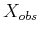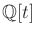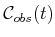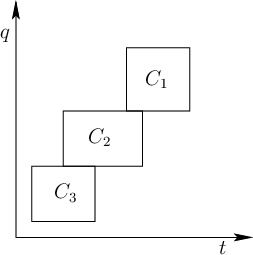#### Combinatorial methods

In some cases, combinatorial methods can be used to solve time-varying problems. If the motion model is algebraic (i.e., expressed with polynomials), thenis semi-algebraic. This enables the application of general planners from Section 6.4, which are based on computational real algebraic geometry. The key issue once again is that the resulting roadmap must be directed with all edges being time-monotonic. For Canny's roadmap algorithm, this requirement seems difficult to ensure. Cylindrical algebraic decomposition is straightforward to adapt, provided that time is chosen as the last variable to be considered in the sequence of projections. This yields polynomials in, andis nicely partitioned into time intervals and time instances. Connections can then be made for a cell of one cylinder to an adjacent cell of a cylinder that occurs later in time.

Ifis polyhedral as depicted in Figure 7.1, then vertical decomposition can be used. It is best to first sweep the plane along the time axis, stopping at the critical times when the linear motion changes. This yields nice sections, which are further decomposed recursively, as explained in Section 6.3.3, and also facilitates the connection of adjacent cells to obtain time-monotonic path segments. It is not too difficult to imagine the approach working for a four-dimensional state space,, for whichis polyhedral as in Section 6.3.3, and time adds the fourth dimension. Again, performing the first sweep with respect to the time axis is preferable.Ifis not decomposed into cylindrical slices over each noncritical time interval, then cell decompositions may still be used, but be careful to correctly connect the cells. Figure 7.2 illustrates the problem, for which transitivity among adjacent cells is broken. This complicates sample point selection for the cells.

Steven M LaValle 2020-08-14